1. /
2. CBSE
3. /
4. Class 06
5. /
6. Mathematics
7. /
8. NCERT Solutions for Class...

# NCERT Solutions for Class 6 Maths Exercise 11.1

NCERT solutions for Maths Algebra## NCERT Solutions for Class 6 Maths Algebra

###### Question 1.Find the rule, which gives the number of matchsticks required to make the following matchsticks patterns. Use a variable to write the rule.

(a)A pattern of letter T as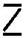(b)A pattern of letter Z as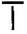(c)A pattern of letter U as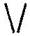(d)A pattern of letter V as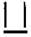(e)A pattern of letter E as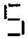(f)A pattern of letter S as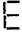(g)A pattern of letter A as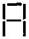(a)Pattern of letter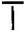= {tex}2n{/tex} (as two matchstick used in each letter)

(b) Pattern of letter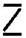= {tex}3n{/tex} (as three matchstick used in each letter)

(c) Pattern of letter= {tex}3n{/tex} (as three matchstick used in each letter)

(d) Pattern of letter= {tex}2n{/tex} (as two matchstick used in each letter)

(e) Pattern of letter= {tex}5n{/tex} (as five matchstick used in each letter)

(f) Pattern of letter= {tex}5n{/tex} (as five matchstick used in each letter)

(g) Pattern of letter= {tex}6n{/tex} (as six matchstick used in each letter)

NCERT Solutions for Class 6 Maths Exercise 11.1

###### Question 2.We already know the rule for the pattern of letter L, C and F. Some of the letters from Q.1 (given above) give us the same rule as that given by L. Which are these? Why does this happen?

Answer: The letter ‘T’ and ‘V’ that has pattern {tex}2n,{/tex} since 2 matchsticks are used in all these letters.

###### Question 3.Cadets are marching in a parade. There are 5 cadets in a row. What is the rule, which gives the number of cadets, given the number of rows? (Use {tex}n{/tex} for the number of rows)

Answer: Number of rows = {tex}n{/tex}

Cadets in each row = 5

Therefore, total number of cadets = {tex}5n{/tex}

NCERT Solutions for Class 6 Maths Exercise 11.1

###### Question 4.If there are 50 mangoes in a box, how will you write the total number of mangoes in terms of the number of boxes? (Use {tex}b{/tex} for the number of boxes)

Answer: Number of boxes = {tex}b{/tex}

Number of mangoes in each box = 50

Therefore, total number of mangoes = {tex}50b{/tex}

###### Question 5.The teacher distributes 5 pencils per student. Can you tell how many pencils are needed, given the number of students? (Use {tex}s{/tex} for the number of students)

Number of students = {tex}s{/tex}

Number of pencils to each student = 5

Therefore, total number of pencils needed are = {tex}5s{/tex}

NCERT Solutions for Class 6 Maths Exercise 11.1

###### Question 6.A bird flies 1 kilometer in one minute. Can you express the distance covered by the bird in terms of its flying time in minutes? (Use {tex}t{/tex} for flying time in minutes)

Answer: Time taken by bird = {tex}t{/tex} minutes

Speed of bird = 1 km per minute

Therefore, Distance covered by bird = speed x time = {tex}1 \times t = t{/tex} km

###### Question 7.Radha is drawing a dot Rangoli (a beautiful pattern of lines joining dots with chalk powder as in figure). She has 8 dots in a row. How many dots will her Rangoli have for {tex}r{/tex} rows? How many dots are there if there are 8 rows? If there are 10 rows?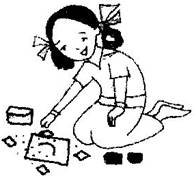Answer: Number of dots in each row = 8 dots

Number of rows = {tex}r{/tex}

Therefore, number of dots = {tex}8r{/tex}

When there are 8 rows, then number of dots = 8 x 8 = 64 dots

When there are 10 rows, then number of dots = 8 x 10 = 80 dots

NCERT Solutions for Class 6 Maths Exercise 11.1

###### Question 8.Leela is Radha’s younger sister. Leela is 4 years younger than Radha. Can you write Leela’s age in terms of Radha’s age? Take Radha’s age to be {tex}x{/tex} years.

Therefore, Leela’s age = {tex}\left( {x – 4} \right){/tex} years

###### Question 9.Mother has made laddus. She gives some laddus to guests and family members; still 5 laddus remain. If the number of laddus mother gave away is {tex}l,{/tex} how many laddus did she make?

Number of laddus remaining = 5

Total number of laddus = {tex}\left( {l + 5} \right){/tex}

NCERT Solutions for Class 6 Maths Exercise 11.1

###### Question 10.Oranges are to be transferred from larger boxes into smaller boxes. When a large box is emptied, the oranges from it fill two smaller boxes and still 10 oranges remain outside. If the number of oranges in a small box are taken to be {tex}x,{/tex} what is the number of oranges in the larger box?

Number of oranges in one box = {tex}x{/tex}

Number of boxes = 2

Therefore, total number of oranges in boxes = {tex}2x{/tex}

Remaining oranges = 10

Thus, number of oranges = {tex}2x + 10{/tex}

NCERT Solutions for Class 6 Maths Exercise 11.1

###### Question 11.

(a) Look at the following matchstick pattern of squares. The squares are not separate. Two neighbouring squares have a common matchstick. Observe the patterns and find the rule that gives the number of matchsticks in terms of the number of squares. (Hint: If you remove the vertical stick at the end, you will get a pattern of Cs.)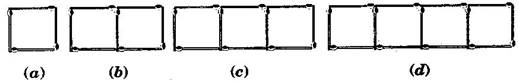(b) Figs. Below gives a matchstick pattern of triangles. As in Exercise 11 (a) above find the general rule that gives the number of matchsticks in terms of the number of triangles.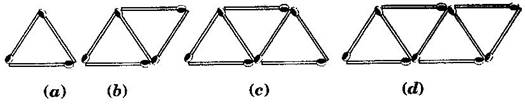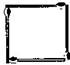4 matchsticks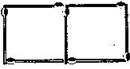7 matchsticks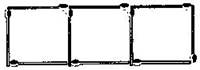10 matchsticks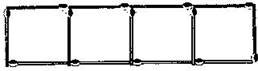13 matchsticks

If we remove 1 from each then they makes table of 3, i.e., 3, 6, 9, 12, ……….

So the required equation = {tex}3x + 1{/tex} , where {tex}x{/tex} is number of squares.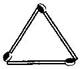3 matchsticks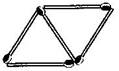7 matchsticks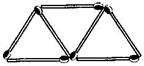10 matchsticks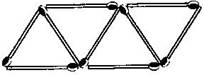13 matchsticks

If we remove 1 from each then they makes table of 2, i.e., 2, 4, 6, 8, ……….

So the required equation = {tex}2x + 1{/tex} , where {tex}x{/tex} is number of triangles.

## NCERT Solutions for Class 6 Maths Exercise 11.1

NCERT Solutions Class 6 Maths PDF (Download) Free from myCBSEguide app and myCBSEguide website. Ncert solution class 6 Maths includes text book solutions from Class 6 Maths Book . NCERT Solutions for CBSE Class 6 Maths have total 14 chapters. 6 Maths NCERT Solutions in PDF for free Download on our website. Ncert Maths class 6 solutions PDF and Maths ncert class 6 PDF solutions with latest modifications and as per the latest CBSE syllabus are only available in myCBSEguide.

## CBSE app for Students

To download NCERT Solutions for Class 6 Maths, Social Science Computer Science, Home Science, Hindi English, Maths Science do check myCBSEguide app or website. myCBSEguide provides sample papers with solution, test papers for chapter-wise practice, NCERT solutions, NCERT Exemplar solutions, quick revision notes for ready reference, CBSE guess papers and CBSE important question papers. Sample Paper all are made available through the best app for CBSE students and myCBSEguide website.Test Generator

Create question paper PDF and online tests with your own name & logo in minutes.myCBSEguide

Question Bank, Mock Tests, Exam Papers, NCERT Solutions, Sample Papers, Notes